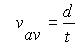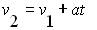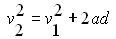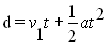## These 5 equations will help you solve Kinematics problems

ONEFinds the average velocity (or speed) when you are given an initial and a final velocity of a moving object.If the object starts from rest the value of v1 is zero

TWOFinds the average velocity (or speed) of a moving object over a definitive distance and time period.

THREEFinds the final velocity of an object undergoing accelelration over a given period of time.If the object starts from rest the value of v1 is zero

FOURFinds the final velocity of an object given its acceleartion and displacement.don't forget to take the square root to get to the answer for final velocity.this equation can be rearranged to solve for the distance or dispalcement that an object travels given its velocities and acceleration.If the object starts from rest the value of v1 is zero

FIVEFinds the total distance traveled by an object over a period of time  if you are given its acceleration.  This is probably the most useful of the 5 equations.memorize this equation!!!!If the object starts from rest the value of v1 is zero